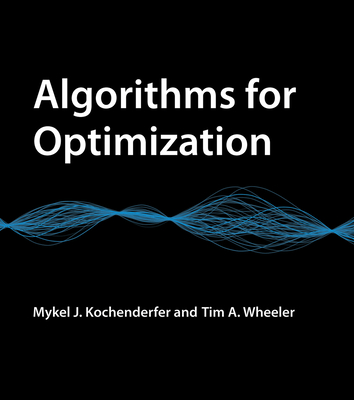### Algorithms for Optimization### Huge savings for students

Each student receives a 50% discount off of most books in the HSG Book Store. During class, please ask the instructor about purchase details.
 List Price: \$85.00 Price: \$42.50 You Save: \$42.50
7A comprehensive introduction to optimization with a focus on practical algorithms for the design of engineering systems.

This book offers a comprehensive introduction to optimization with a focus on practical algorithms. The book approaches optimization from an engineering perspective, where the objective is to design a system that optimizes a set of metrics subject to constraints. Readers will learn about computational approaches for a range of challenges, including searching high-dimensional spaces, handling problems where there are multiple competing objectives, and accommodating uncertainty in the metrics. Figures, examples, and exercises convey the intuition behind the mathematical approaches. The text provides concrete implementations in the Julia programming language.

Topics covered include derivatives and their generalization to multiple dimensions; local descent and first- and second-order methods that inform local descent; stochastic methods, which introduce randomness into the optimization process; linear constrained optimization, when both the objective function and the constraints are linear; surrogate models, probabilistic surrogate models, and using probabilistic surrogate models to guide optimization; optimization under uncertainty; uncertainty propagation; expression optimization; and multidisciplinary design optimization. Appendixes offer an introduction to the Julia language, test functions for evaluating algorithm performance, and mathematical concepts used in the derivation and analysis of the optimization methods discussed in the text. The book can be used by advanced undergraduates and graduate students in mathematics, statistics, computer science, any engineering field, (including electrical engineering and aerospace engineering), and operations research, and as a reference for professionals.

MIT Press